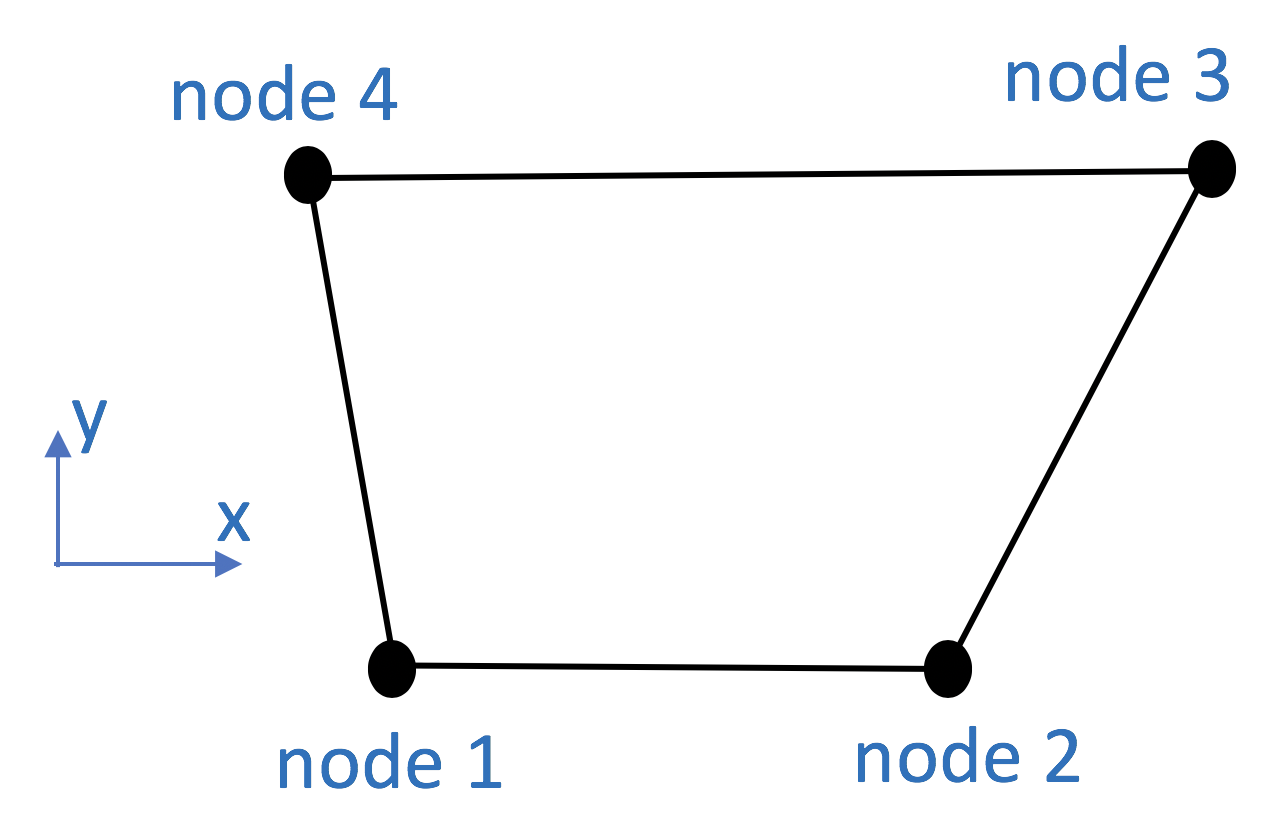This command is used to construct a FourNodeQuad element object which uses a bilinear isoparametric formulation.

Command

element quad \$eleTag \$iNode \$jNode \$kNode \$lNode \$thick \$type \$matTag <\$pressure \$rho \$b1 \$b2>

Argument

Type

Description

\$eleTag

integer

unique element object tag

\$iNode \$jNode \$kNode \$lNode

integer four nodes defining element boundaries

input in counter-clockwise order around the element.

\$thick

float

element thickness

\$type

string

string representing material behavior. The type parameter can be either “PlaneStrain” or “PlaneStress.”

\$matTag

integer

tag of nDMaterial

\$pressure

float

surface pressure (optional: default = 0.0)

\$rho

float

element mass density (per unit volume) from which a lumped element mass matrix is computed (optional: default=0.0)

\$b1 \$b2

float

constant body forces defined in the isoparametric domain (optional: default=0.0)Fig. 3.1.9.12 Quad Element Node Numbering

Note

The optional arguments must either be all specified or none specified.

Consistent nodal loads are computed from the pressure and body forces.

The valid queries to a Quad element when creating an ElementRecorder object are ‘forces’, ‘stresses,’ and ‘material \$matNum matArg1 matArg2 …’ Where \$matNum refers to the material object at the integration point corresponding to the node numbers in the isoparametric domain.

Example

The following example constructs a quad element for use in a plane stress problem with tag 1 between nodes 1, 2, 3, 4 with an nDMaterial of tag 1.

1. Tcl Code

```element stdBrick 1 1 2 3 4 "PlaneStress" 1
```
1. Python Code

```element('quad',1,1,2,3,4, 'PlaneStress', 1, b1, b2, b3)
```

Code Developed by: Michael H. Scott